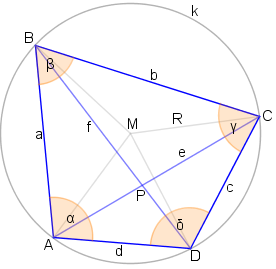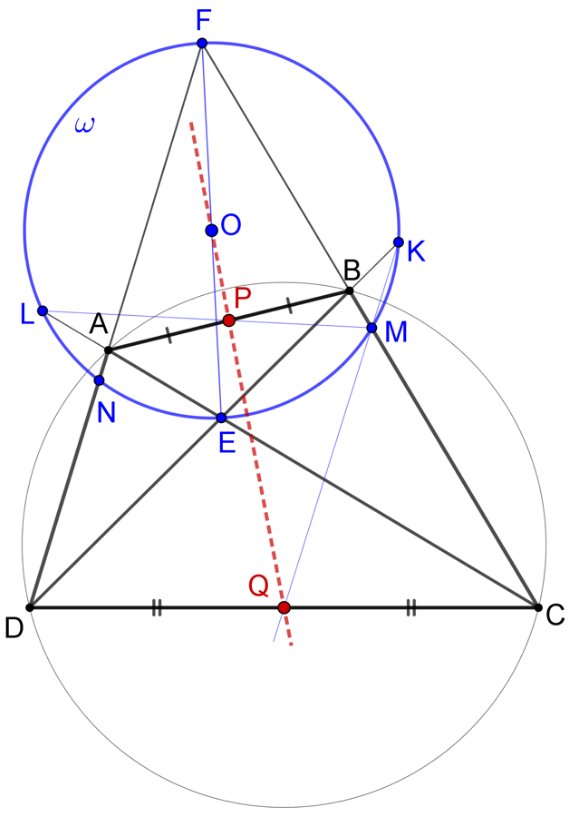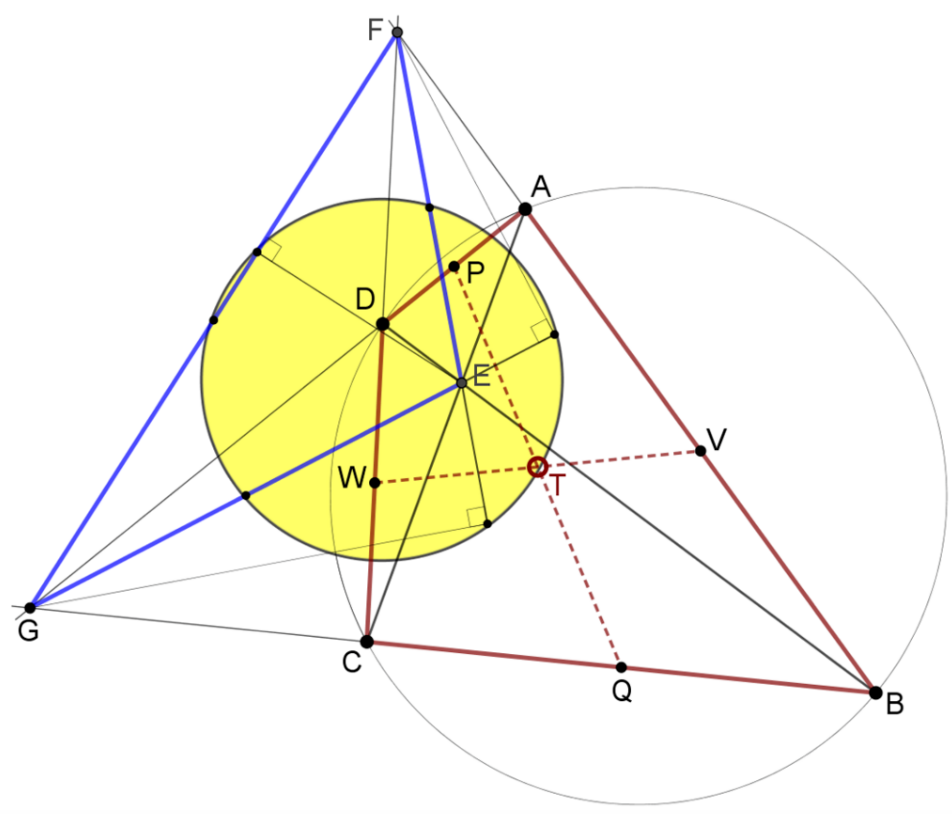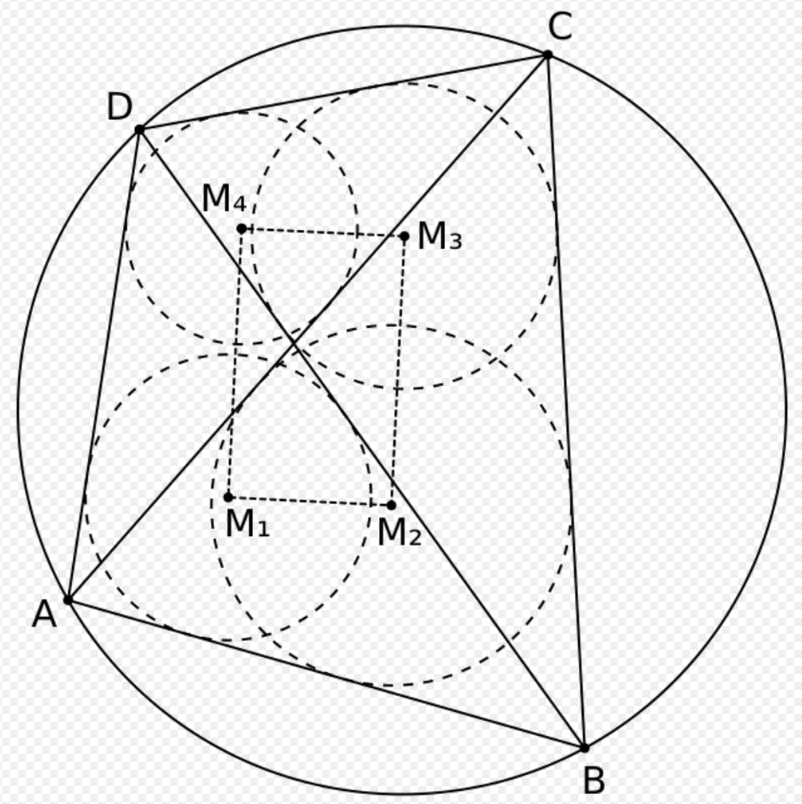Submitted Successfully!
Thank you for your contribution! You can also upload a video entry or images related to this topic.
Ver. Summary Created by Modification Content Size Created at Operation
1 handwiki -- 2544 2022-10-27 01:38:48

Do you have a full video?

# Confirm

Are you sure to Delete?
Cite
Li, H. Cyclic Quadrilateral. Encyclopedia. Available online: https://encyclopedia.pub/entry/31529 (accessed on 29 November 2023).
Li H. Cyclic Quadrilateral. Encyclopedia. Available at: https://encyclopedia.pub/entry/31529. Accessed November 29, 2023.
Li, Handwiki. "Cyclic Quadrilateral" Encyclopedia, https://encyclopedia.pub/entry/31529 (accessed November 29, 2023).
Li, H.(2022, October 27). Cyclic Quadrilateral. In Encyclopedia. https://encyclopedia.pub/entry/31529
Li, Handwiki. "Cyclic Quadrilateral." Encyclopedia. Web. 27 October, 2022.

## 1. Special Cases

Any square, rectangle, isosceles trapezoid, or antiparallelogram is cyclic. A kite is cyclic if and only if it has two right angles. A bicentric quadrilateral is a cyclic quadrilateral that is also tangential and an ex-bicentric quadrilateral is a cyclic quadrilateral that is also ex-tangential. A harmonic quadrilateral is a cyclic quadrilateral in which the product of the lengths of opposite sides are equal.

## 2. Characterizations### 2.1. Circumcenter

A convex quadrilateral is cyclic if and only if the four perpendicular bisectors to the sides are concurrent. This common point is the circumcenter.

### 2.2. Supplementary Angles

A convex quadrilateral ABCD is cyclic if and only if its opposite angles are supplementary, that is 

$\displaystyle{ \alpha + \gamma = \beta + \delta = \pi \ (= 180^{\circ}). }$

The direct theorem was Proposition 22 in Book 3 of Euclid's Elements. Equivalently, a convex quadrilateral is cyclic if and only if each exterior angle is equal to the opposite interior angle.

### 2.3. Angles Between Sides and Diagonals

A convex quadrilateral ABCD is cyclic if and only if an angle between a side and a diagonal is equal to the angle between the opposite side and the other diagonal. That is, for example,

$\displaystyle{ \angle ACB = \angle ADB. }$

### 2.4. Intersection of DiagonalsABCD is a cyclic quadrilateral. E is the point of intersection of the diagonals and F is the point of intersection of the extensions of sides BC and AD. $\displaystyle{ \omega }$ is a circle whose diameter is the segment, EF. P and Q are Pascal points formed by the circle $\displaystyle{ \omega }$. https://handwiki.org/wiki/index.php?curid=1197491

Another necessary and sufficient conditions for a convex quadrilateral ABCD to be cyclic are: let E be the point of intersection of the diagonals, let F be the intersection point of the extensions of the sides AD and BC, let $\displaystyle{ \omega }$ be a circle whose diameter is the segment, EF, and let P and Q be Pascal points on sides AB and CD formed by the circle $\displaystyle{ \omega }$.
(1) ABCD is a cyclic quadrilateral if and only if points P and Q are collinear with the center O, of circle $\displaystyle{ \omega }$.
(2) ABCD is a cyclic quadrilateral if and only if points P and Q are the midpoints of sides AB and CD.

If two lines, one containing segment AC and the other containing segment BD, intersect at P, then the four points A, B, C, D are concyclic if and only if

$\displaystyle{ \displaystyle AP\cdot PC = BP\cdot PD. }$

The intersection P may be internal or external to the circle. In the former case, the cyclic quadrilateral is ABCD, and in the latter case, the cyclic quadrilateral is ABDC. When the intersection is internal, the equality states that the product of the segment lengths into which P divides one diagonal equals that of the other diagonal. This is known as the intersecting chords theorem since the diagonals of the cyclic quadrilateral are chords of the circumcircle.

### 2.5. Ptolemy's Theorem

Ptolemy's theorem expresses the product of the lengths of the two diagonals e and f of a cyclic quadrilateral as equal to the sum of the products of opposite sides::p.25

$\displaystyle{ \displaystyle ef = ac + bd. }$

The converse is also true. That is, if this equation is satisfied in a convex quadrilateral, then a cyclic quadrilateral is formed.

### 2.6. Diagonal TriangleABCD is a cyclic quadrilateral. EFG is the diagonal triangle of ABCD. The point T of intersection of the bimedians of ABCD belongs to the nine-point circle of EFG. https://handwiki.org/wiki/index.php?curid=1808537

In a convex quadrilateral ABCD, let EFG be the diagonal triangle of ABCD and let $\displaystyle{ \omega }$ be the nine-point circle of EFG. ABCD is cyclic if and only if the point of intersection of the bimedians of ABCD belongs to the nine-point circle $\displaystyle{ \omega }$.

### 2.7. Tangent Products

A convex quadrilateral ABCD is cyclic if and only if

$\displaystyle{ \tan{\frac{\alpha}{2}}\tan{\frac{\gamma}{2}}=\tan{\frac{\beta}{2}}\tan{\frac{\delta}{2}}=1. }$

## 3. Area

The area K of a cyclic quadrilateral with sides a, b, c, d is given by Brahmagupta's formula:p.24

$\displaystyle{ K=\sqrt{(s-a)(s-b)(s-c)(s-d)} \, }$

where s, the semiperimeter, is s = 1/2(a + b + c + d). This is a corollary of Bretschneider's formula for the general quadrilateral, since opposite angles are supplementary in the cyclic case. If also d = 0, the cyclic quadrilateral becomes a triangle and the formula is reduced to Heron's formula.

The cyclic quadrilateral has maximal area among all quadrilaterals having the same sequence of side lengths. This is another corollary to Bretschneider's formula. It can also be proved using calculus.

Four unequal lengths, each less than the sum of the other three, are the sides of each of three non-congruent cyclic quadrilaterals, which by Brahmagupta's formula all have the same area. Specifically, for sides a, b, c, and d, side a could be opposite any of side b, side c, or side d.

The area of a cyclic quadrilateral with successive sides a, b, c, d and angle B between sides a and b can be expressed as:p.25

$\displaystyle{ K = \tfrac{1}{2}(ab+cd)\sin{B} }$

or:p.26

$\displaystyle{ K = \tfrac{1}{2}(ac+bd)\sin{\theta} }$

where θ is either angle between the diagonals. Provided A is not a right angle, the area can also be expressed as:p.26

$\displaystyle{ K = \tfrac{1}{4}(a^2-b^2-c^2+d^2)\tan{A}. }$

Another formula is:p.83

$\displaystyle{ \displaystyle K=2R^2\sin{A}\sin{B}\sin{\theta} }$

where R is the radius of the circumcircle. As a direct consequence,

$\displaystyle{ K\le 2R^2 }$

where there is equality if and only if the quadrilateral is a square.

## 4. Diagonals

In a cyclic quadrilateral with successive vertices A, B, C, D and sides a = AB, b = BC, c = CD, and d = DA, the lengths of the diagonals p = AC and q = BD can be expressed in terms of the sides as:p.25,:p. 84

$\displaystyle{ p = \sqrt{\frac{(ac+bd)(ad+bc)}{ab+cd}} }$ and $\displaystyle{ q = \sqrt{\frac{(ac+bd)(ab+cd)}{ad+bc}} }$

so showing Ptolemy's theorem

$\displaystyle{ pq = ac+bd. }$

According to Ptolemy's second theorem,:p.25,

$\displaystyle{ \frac {p}{q}= \frac{ad+bc}{ab+cd} }$

using the same notations as above.

For the sum of the diagonals we have the inequality:p.123,#2975

$\displaystyle{ p+q\ge 2\sqrt{ac+bd}. }$

Equality holds if and only if the diagonals have equal length, which can be proved using the AM-GM inequality.

Moreover,:p.64,#1639

$\displaystyle{ (p+q)^2 \leq (a+c)^2+(b+d)^2. }$

In any convex quadrilateral, the two diagonals together partition the quadrilateral into four triangles; in a cyclic quadrilateral, opposite pairs of these four triangles are similar to each other.

If M and N are the midpoints of the diagonals AC and BD, then

$\displaystyle{ \frac{MN}{EF}=\frac{1}{2}\left |\frac{AC}{BD}-\frac{BD}{AC}\right| }$

where E and F are the intersection points of the extensions of opposite sides.

If ABCD is a cyclic quadrilateral where AC meets BD at E, then

$\displaystyle{ \frac{AE}{CE}=\frac{AB}{CB}\cdot\frac{AD}{CD}. }$

A set of sides that can form a cyclic quadrilateral can be arranged in any of three distinct sequences each of which can form a cyclic quadrilateral of the same area in the same circumcircle (the areas being the same according to Brahmagupta's area formula). Any two of these cyclic quadrilaterals have one diagonal length in common.:p. 84

## 5. Angle Formulas

For a cyclic quadrilateral with successive sides a, b, c, d, semiperimeter s, and angle A between sides a and d, the trigonometric functions of A are given by

$\displaystyle{ \cos A = \frac{a^2 + d^2 - b^2 - c^2}{2(ad + bc)}, }$
$\displaystyle{ \sin A = \frac{2\sqrt{(s-a)(s-b)(s-c)(s-d)}}{(ad+bc)}, }$
$\displaystyle{ \tan \frac{A}{2} = \sqrt{\frac{(s-a)(s-d)}{(s-b)(s-c)}}. }$

The angle θ between the diagonals satisfies:p.26

$\displaystyle{ \tan \frac{\theta}{2} = \sqrt{\frac{(s-b)(s-d)}{(s-a)(s-c)}}. }$

If the extensions of opposite sides a and c intersect at an angle φ, then

$\displaystyle{ \cos{\frac{\varphi}{2}}=\sqrt{\frac{(s-b)(s-d)(b+d)^2}{(ab+cd)(ad+bc)}} }$

where s is the semiperimeter.:p.31

A cyclic quadrilateral with successive sides a, b, c, d and semiperimeter s has the circumradius (the radius of the circumcircle) given by

$\displaystyle{ R=\frac{1}{4} \sqrt{\frac{(ab+cd)(ac+bd)(ad+bc)}{(s-a)(s-b)(s-c)(s-d)}}. }$

This was derived by the Indian mathematician Vatasseri Parameshvara in the 15th century.

Using Brahmagupta's formula, Parameshvara's formula can be restated as

$\displaystyle{ 4KR=\sqrt{(ab+cd)(ac+bd)(ad+bc)} }$

where K is the area of the cyclic quadrilateral.

## 7. Anticenter and Collinearities

Four line segments, each perpendicular to one side of a cyclic quadrilateral and passing through the opposite side's midpoint, are concurrent.:p.131; These line segments are called the maltitudes, which is an abbreviation for midpoint altitude. Their common point is called the anticenter. It has the property of being the reflection of the circumcenter in the "vertex centroid". Thus in a cyclic quadrilateral, the circumcenter, the "vertex centroid", and the anticenter are collinear.

If the diagonals of a cyclic quadrilateral intersect at P, and the midpoints of the diagonals are M and N, then the anticenter of the quadrilateral is the orthocenter of triangle MNP.

## 8. Other PropertiesJapanese theorem. https://handwiki.org/wiki/index.php?curid=2044785
• In a cyclic quadrilateral ABCD, the incenters M1, M2, M3, M4 (see the figure to the right) in triangles DAB, ABC, BCD, and CDA are the vertices of a rectangle. This is one of the theorems known as the Japanese theorem. The orthocenters of the same four triangles are the vertices of a quadrilateral congruent to ABCD, and the centroids in those four triangles are vertices of another cyclic quadrilateral.
• In a cyclic quadrilateral ABCD with circumcenter O, let P be the point where the diagonals AC and BD intersect. Then angle APB is the arithmetic mean of the angles AOB and COD. This is a direct consequence of the inscribed angle theorem and the exterior angle theorem.
• There are no cyclic quadrilaterals with rational area and with unequal rational sides in either arithmetic or geometric progression.
• If a cyclic quadrilateral has side lengths that form an arithmetic progression the quadrilateral is also ex-bicentric.
• If the opposite sides of a cyclic quadrilateral are extended to meet at E and F, then the internal angle bisectors of the angles at E and F are perpendicular.

A Brahmagupta quadrilateral is a cyclic quadrilateral with integer sides, integer diagonals, and integer area. All Brahmagupta quadrilaterals with sides a, b, c, d, diagonals e, f, area K, and circumradius R can be obtained by clearing denominators from the following expressions involving rational parameters t, u, and v:

$\displaystyle{ a=[t(u+v)+(1-uv)][u+v-t(1-uv)] }$
$\displaystyle{ b=(1+u^2)(v-t)(1+tv) }$
$\displaystyle{ c=t(1+u^2)(1+v^2) }$
$\displaystyle{ d=(1+v^2)(u-t)(1+tu) }$
$\displaystyle{ e=u(1+t^2)(1+v^2) }$
$\displaystyle{ f=v(1+t^2)(1+u^2) }$
$\displaystyle{ K=uv[2t(1-uv)-(u+v)(1-t^2)][2(u+v)t+(1-uv)(1-t^2)] }$
$\displaystyle{ 4R=(1+u^2)(1+v^2)(1+t^2). }$

## 10. Orthodiagonal Case

For a cyclic quadrilateral that is also orthodiagonal (has perpendicular diagonals), suppose the intersection of the diagonals divides one diagonal into segments of lengths p1 and p2 and divides the other diagonal into segments of lengths q1 and q2. Then (the first equality is Proposition 11 in Archimedes' Book of Lemmas)

$\displaystyle{ D^2=p_1^2+p_2^2+q_1^2+q_2^2=a^2+c^2=b^2+d^2 }$

where D is the diameter of the circumcircle. This holds because the diagonals are perpendicular chords of a circle. These equations imply that the circumradius R can be expressed as

$\displaystyle{ R=\tfrac{1}{2}\sqrt{p_1^2+p_2^2+q_1^2+q_2^2} }$

or, in terms of the sides of the quadrilateral, as

$\displaystyle{ R=\tfrac{1}{2}\sqrt{a^2+c^2}=\tfrac{1}{2}\sqrt{b^2+d^2}. }$

It also follows that

$\displaystyle{ a^2+b^2+c^2+d^2=8R^2. }$

Thus, according to Euler's quadrilateral theorem, the circumradius can be expressed in terms of the diagonals p and q, and the distance x between the midpoints of the diagonals as

$\displaystyle{ R=\sqrt{\frac{p^2+q^2+4x^2}{8}}. }$

A formula for the area K of a cyclic orthodiagonal quadrilateral in terms of the four sides is obtained directly when combining Ptolemy's theorem and the formula for the area of an orthodiagonal quadrilateral. The result is:p.222

$\displaystyle{ K=\tfrac{1}{2}(ac+bd). }$

### 10.2. Other Properties

• In a cyclic orthodiagonal quadrilateral, the anticenter coincides with the point where the diagonals intersect.
• Brahmagupta's theorem states that for a cyclic quadrilateral that is also orthodiagonal, the perpendicular from any side through the point of intersection of the diagonals bisects the opposite side.
• If a cyclic quadrilateral is also orthodiagonal, the distance from the circumcenter to any side equals half the length of the opposite side.
• In a cyclic orthodiagonal quadrilateral, the distance between the midpoints of the diagonals equals the distance between the circumcenter and the point where the diagonals intersect.

In spherical geometry, a spherical quadrilateral formed from four intersecting greater circles is cyclic if and only if the summations of the opposite angles are equal, i.e., α + γ = β + δ for consecutive angles α, β, γ, δ of the quadrilateral. One direction of this theorem was proved by I. A. Lexell in 1786. Lexell showed that in a spherical quadrilateral inscribed in a small circle of a sphere the sums of opposite angles are equal, and that in the circumscribed quadrilateral the sums of opposite sides are equal. The first of these theorems is the spherical analogue of a plane theorem, and the second theorem is its dual, that is, the result of interchanging great circles and their poles. Kiper et al. proved a converse of the theorem: If the summations of the opposite sides are equal in a spherical quadrilateral, then there exists an inscribing circle for this quadrilateral.

### References

1. Usiskin, Zalman; Griffin, Jennifer; Witonsky, David; Willmore, Edwin (2008), "10. Cyclic quadrilaterals", The Classification of Quadrilaterals: A Study of Definition, Research in mathematics education, IAP, pp. 63–65, ISBN 978-1-59311-695-8, https://books.google.com/books?id=ZkoUR5lRwdcC&pg=PA63
2. Fraivert, David; Sigler, Avi; Stupel, Moshe (2019), "Necessary and sufficient properties for a cyclic quadrilateral", International Journal of Mathematical Education in Science and Technology: 1–26, doi:10.1080/0020739X.2019.1683772, https://www.tandfonline.com/eprint/G7K9HXIRZKCW9GQCPEZ6/full?target=10.1080/0020739X.2019.1683772
3. Joyce, D. E. (June 1997), "Book 3, Proposition 22", Euclid's Elements, Clark University, http://aleph0.clarku.edu/~djoyce/java/elements/bookIII/propIII22.html
4. Andreescu, Titu; Enescu, Bogdan (2004), "2.3 Cyclic quads", Mathematical Olympiad Treasures, Springer, pp. 44–46, 50, ISBN 978-0-8176-4305-8, https://books.google.com/books?id=mwUHJpvLOPsC&pg=PA44
5. Bradley, Christopher J. (2007), The Algebra of Geometry: Cartesian, Areal and Projective Co-Ordinates, Highperception, p. 179, ISBN 978-1906338008, OCLC 213434422  http://www.worldcat.org/oclc/213434422
6. Durell, C. V.; Robson, A. (2003) , Advanced Trigonometry, Courier Dover, ISBN 978-0-486-43229-8, https://books.google.com/books?id=3iYbExAsepEC
7. Fraivert, David (July 2019). "New points that belong to the nine-point circle". The Mathematical Gazette 103 (557): 222–232. doi:10.1017/mag.2019.53.  https://dx.doi.org/10.1017%2Fmag.2019.53
8. Fraivert, David (2018). "New applications of method of complex numbers in the geometry of cyclic quadrilaterals". International Journal of Geometry 7 (1): 5–16. https://ijgeometry.com/wp-content/uploads/2018/04/5-16.pdf.
9. Hajja, Mowaffaq (2008), "A condition for a circumscriptible quadrilateral to be cyclic", Forum Geometricorum 8: 103–6, http://forumgeom.fau.edu/FG2008volume8/FG200814.pdf
10. Peter, Thomas (September 2003), "Maximizing the area of a quadrilateral", The College Mathematics Journal 34 (4): 315–6, doi:10.2307/3595770  https://dx.doi.org/10.2307%2F3595770
11. Coxeter, Harold Scott MacDonald; Greitzer, Samuel L. (1967), "3.2 Cyclic Quadrangles; Brahmagupta's formula", Geometry Revisited, Mathematical Association of America, pp. 57, 60, ISBN 978-0-88385-619-2, https://books.google.com/books?id=VdAM58ksvcIC&pg=PA57
12. Prasolov, Viktor, Problems in plane and solid geometry: v.1 Plane Geometry, http://students.imsa.edu/~tliu/Math/planegeo.pdf, retrieved November 6, 2011
13. Alsina, Claudi; Nelsen, Roger (2009), "4.3 Cyclic, tangential, and bicentric quadrilaterals", When Less is More: Visualizing Basic Inequalities, Mathematical Association of America, p. 64, ISBN 978-0-88385-342-9, https://books.google.com/books?id=U1ovBsSRNscC&pg=PA64
14. Alsina, Claudi; Nelsen, Roger B. (2007), "On the diagonals of a cyclic quadrilateral", Forum Geometricorum 7: 147–9, http://forumgeom.fau.edu/FG2007volume7/FG200720.pdf
15. Johnson, Roger A., Advanced Euclidean Geometry, Dover Publ., 2007 (orig. 1929).
16. Inequalities proposed in "Crux Mathematicorum", 2007, .
17. "ABCD is a cyclic quadrilateral. Let M, N be midpoints of diagonals AC, BD respectively...". Art of Problem Solving. 2010. http://www.artofproblemsolving.com/Forum/viewtopic.php?f=46&t=350179.
18. A. Bogomolny, An Identity in (Cyclic) Quadrilaterals, Interactive Mathematics Miscellany and Puzzles, , Accessed 18 March 2014.
19. Siddons, A. W.; Hughes, R. T. (1929), Trigonometry, Cambridge University Press, p. 202, OCLC 429528983  http://www.worldcat.org/oclc/429528983
20. Hoehn, Larry (March 2000), "Circumradius of a cyclic quadrilateral", Mathematical Gazette 84 (499): 69–70, doi:10.2307/3621477  https://dx.doi.org/10.2307%2F3621477
21. Altshiller-Court, Nathan (2007) , College Geometry: An Introduction to the Modern Geometry of the Triangle and the Circle (2nd ed.), Courier Dover, pp. 131, 137–8, ISBN 978-0-486-45805-2, OCLC 78063045  http://www.worldcat.org/oclc/78063045
22. Honsberger, Ross (1995), "4.2 Cyclic quadrilaterals", Episodes in Nineteenth and Twentieth Century Euclidean Geometry, New Mathematical Library, 37, Cambridge University Press, pp. 35–39, ISBN 978-0-88385-639-0, https://books.google.com/books?id=6oduPgvOAhwC&pg=PA35
23. Weisstein, Eric W.. "Maltitude". http://mathworld.wolfram.com/Maltitude.html.
24. Buchholz, R. H.; MacDougall, J. A. (1999), "Heron quadrilaterals with sides in arithmetic or geometric progression", Bulletin of the Australian Mathematical Society 59 (2): 263–9, doi:10.1017/S0004972700032883  https://dx.doi.org/10.1017%2FS0004972700032883
25. Sastry, K.R.S. (2002). "Brahmagupta quadrilaterals". Forum Geometricorum 2: 167–173. http://forumgeom.fau.edu/FG2002volume2/FG200221.pdf.
26. Posamentier, Alfred S.; Salkind, Charles T. (1970), "Solutions: 4-23 Prove that the sum of the squares of the measures of the segments made by two perpendicular chords is equal to the square of the measure of the diameter of the given circle.", Challenging Problems in Geometry (2nd ed.), Courier Dover, pp. 104–5, ISBN 978-0-486-69154-1, https://books.google.com/books?id=ld-_Puq62mcC&pg=PA104
27. Josefsson, Martin (2016), "Properties of Pythagorean quadrilaterals", The Mathematical Gazette 100 (July): 213–224, doi:10.1017/mag.2016.57 . https://dx.doi.org/10.1017%2Fmag.2016.57
28. Wimmer, Lienhard (2011). "Cyclic polygons in non-Euclidean geometry". Elemente der Mathematik 66 (2): 74–82.
29. Lexell, A. J. (1786). "De proprietatibus circulorum in superficie sphaerica descriptorum". Acta Acad. Sci. Petropol. 6 (1): 58–103.
30. Rosenfeld, B. A. (1988). A History of Non-Euclidean Geometry - Springer. Studies in the History of Mathematics and Physical Sciences. 12. doi:10.1007/978-1-4419-8680-1. ISBN 978-1-4612-6449-1.  https://dx.doi.org/10.1007%2F978-1-4419-8680-1
31. Kiper, Gökhan; Söylemez, Eres (2012-05-01). "Homothetic Jitterbug-like linkages". Mechanism and Machine Theory 51: 145–158. doi:10.1016/j.mechmachtheory.2011.11.014.  https://dx.doi.org/10.1016%2Fj.mechmachtheory.2011.11.014
More
Information
Subjects:
Contributor MDPI registered users' name will be linked to their SciProfiles pages. To register with us, please refer to https://encyclopedia.pub/register :
View Times: 1563
Entry Collection:
Revision: 1 time (View History)
Update Date: 27 Oct 2022
1000/1000